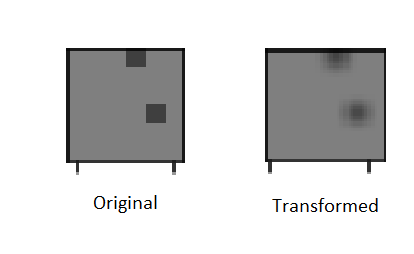# Trasforming a tensor to different size

Hello, I have a 4D tensor of size e.g. (batch_size=10, channels=10, height=6, width=6). I want to resize each (6x6) channel to (30x30). I want to conserve the pixel values as much as possible during resizing, maybe by interpolation or so.

How can I do it for all the channels and images at once instead of one by one with torchvision.transforms?

This might work

``````import torch
from torchvision import transforms
transform = transforms.Compose([
transforms.Resize((30,30)),
])
x = torch.ones(10, 10, 6, 6)
y = transform(x)
print(y.shape)
``````

Output -

``````torch.Size([10, 10, 30, 30])
``````